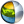Cg 3.1 Toolkit Documentation Cg / Standard Library / pack

## Name

pack - packs mutiple values into a single 32-bit result

## Synopsis

```float pack_2half(float2 a);
float pack_2half(half2 a);
float pack_2ushort(float2 a);
float pack_2ushort(half2 a);
float pack_4byte(float4 a);
float pack_4byte(half4 a);
float pack_4ubyte(float4 a);
float pack_4ubyte(half4 a);
```

a
Value to pack

## Description

pack_2half converts the components of a into a pair of 16-bit floating point values. The two converted components are then packed into a single 32-bit result.

pack_2ushort converts the components of a into a pair of 16-bit unsigned integers. The two converted components are then packed into a single 32-bit return value.

pack_4byte converts the four components of a into 8-bit signed integers. The signed integers are such that a representation with all bits set to 0 corresponds to the value -(128/127), and a representation with all bits set to 1 corresponds to +(127/127). The four signed integers are then packed into a single 32-bit result.

pack_4ubyte converts the four components of a into 8-bit unsigned integers. The unsigned integers are such that a representation with all bits set to 0 corresponds to 0.0, and a representation with all bits set to 1 corresponds to 1.0. The four unsigned integers are then packed into a single 32-bit result.

## Reference Implementation

pack_2half could be implemented this way:

```float pack_2half(float2 a)
{
float result;
return result = (((half)a.y) << 16) | (half)a.x;
}
```

pack_2ushort could be implemented this way:

```float pack_2ushort(float2 a)
{
float result;
ushort.x = round(65535.0 * clamp(a.x, 0.0, 1.0));
ushort.y = round(65535.0 * clamp(a.y, 0.0, 1.0));
result = (ushort.y << 16) | ushort.y;
return result;
}
```

pack_4byte could be implemented this way:

```float pack_2half(half2 a)
{
float result;
ub.y = round(127 * clamp(a.y, -128/127, 127/127) + 128);
ub.z = round(127 * clamp(a.z, -128/127, 127/127) + 128);
ub.w = round(127 * clamp(a.w, -128/127, 127/127) + 128);
return result = (ub.w << 24) | (ub.z << 16) | (ub.y << 8) | ub.x;
}
```

pack_4ubyte could be implemented this way:

```float pack_4ubyte(float4 a)
{
float result;
ub.x = round(255.0 * clamp(a.x, 0.0, 1.0));
ub.y = round(255.0 * clamp(a.y, 0.0, 1.0));
ub.z = round(255.0 * clamp(a.z, 0.0, 1.0));
ub.w = round(255.0 * clamp(a.w, 0.0, 1.0));
result = (ub.w << 24) | (ub.z << 16) | (ub.y << 8) | ub.x;
return result;
}
```

## Profile Support

pack is supported in fp30, fp40, gp4, gp5| Cg Toolkit | Download | Release Archive | Profiles | Reference | Books | Discussions |